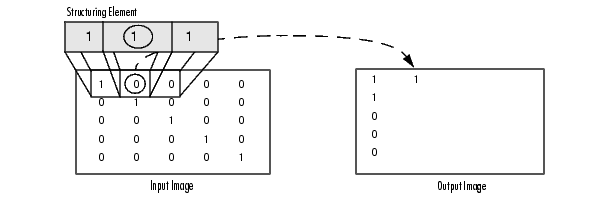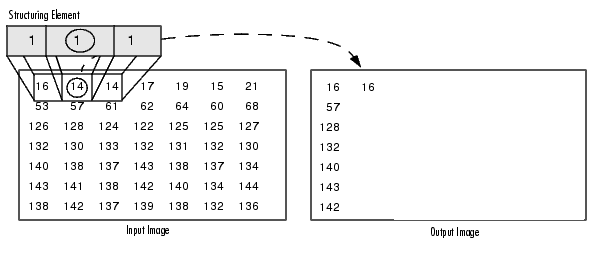Image Processing Toolbox User's GuideUnderstanding Dilation and Erosion

In the morphological dilation and erosion operations, the state of any given pixel in the output image is determined by applying a rule to the corresponding pixel and its neighbors in the input image. The rule used to process the pixels defines the operation as a dilation or an erosion. This table lists the rules for both dilation and erosion.

 Operation Rule Dilation The value of the output pixel is the maximum value of all the pixels in the input pixel's neighborhood. In a binary image, if any of the pixels is set to the value 1, the output pixel is set to 1. Erosion The value of the output pixel is the minimum value of all the pixels in the input pixel's neighborhood. In a binary image, if any of the pixels is set to 0, the output pixel is set to 0.

The following figure illustrates the dilation of a binary image. Note how the structuring element defines the neighborhood of the pixel of interest, which is circled. (See Structuring Elements for more information.) The dilation function applies the appropriate rule to the pixels in the neighborhood and assigns a value to the corresponding pixel in the output image. In the figure, the morphological dilation function sets the value of the output pixel to 1 because one of the elements in the neighborhood defined by the structuring element is on.Morphological Dilation of a Binary Image

The following figure illustrates this processing for a grayscale image. The figure shows the processing of a particular pixel in the input image. Note how the function applies the rule to the input pixel's neighborhood and uses the highest value of all the pixels in the neighborhood as the value of the corresponding pixel in the output image.Morphological Dilation of a Grayscale Image

Processing Pixels at Image Borders (Padding Behavior)

Morphological functions position the origin of the structuring element, its center element, over the pixel of interest in the input image. For pixels at the edge of an image, parts of the neighborhood defined by the structuring element can extend past the border of the image.

To process border pixels, the morphological functions assign a value to these undefined pixels, as if the functions had padded the image with additional rows and columns. The value of these padding pixels varies for dilation and erosion operations. The following table describes the padding rules for dilation and erosion for both binary and grayscale images.

 Operation Rule Dilation Pixels beyond the image border are assigned the minimum value afforded by the data type.For binary images, these pixels are assumed to be set to 0. For grayscale images, the minimum value for uint8 images is 0. Erosion Pixels beyond the image border are assigned the maximum value afforded by the data type.For binary images, these pixels are assumed to be set to 1. For grayscale images, the maximum value for uint8 images is 255.

 Note    By using the minimum value for dilation operations and the maximum value for erosion operations, the toolbox avoids border effects, where regions near the borders of the output image do not appear to be homogeneous with the rest of the image. For example, if erosion padded with a minimum value, eroding an image would result in a black border around the edge of the output image.Dilation and Erosion Structuring Elements